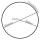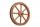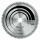Circle r,D

Calculate the diameter and radius of the circle if it has length 52.45 cm.

Result

r =  8.348 cm
D =  16.695 cm

Solution:Leave us a comment of example and its solution (i.e. if it is still somewhat unclear...):Be the first to comment!Next similar examples:

1. Circle - simpleCalculate the area of a circle in dm2, if its circumference is 31.4 cm.
2. MineWheel in traction tower has a diameter 5 m. How many meters will perform an elevator cabin if wheel rotates in the same direction 49 times?
3. FlowerbedIn the park there is a large circular flowerbed with a diameter of 12 m. Jakub circulated him ten times and the smaller Vojtoseven times. How many meters each went by and how many meters did Jakub run more than Vojta?
4. WellRope with a bucket is fixed on the shaft with the wheel. The shaft has a diameter 50 cm. How many meters will drop bucket when the wheels turn 15 times?
5. Circumference - a simpleWhat is the ratio of the circumference of any circle and its diameter? Write the result as a real number rounded to 2 decimal places.
6. Circle - simpleThe circumference of a circle is 930 mm. How long in mm is its diameter?
7. Wheel diameterA 1m diameter wheel rolled along a 100m long track. How many times did it turn?
8. SawImagine that the saw blade with diameter 40 cm has one tooth colored with a different color. How long is path describing by the tip of the tooth after 15 minutes, if the blade is rotated 13365 times?
9. CircleWhat is the radius of the circle whose perimeter is 6 cm?
10. Bicycle wheelAfter driving 157 m bicycle wheel rotates 100 times. What is the radius of the wheel in cm?
11. Two circlesTwo circles with a radius 4 cm and 3 cm have a center distance 0.5cm. How many common points have these circles?
12. Clock handsThe second hand has a length of 1.5 cm. How long does the endpoint of this hand travel in one day?
13. Percents - easyHow many percent is 432 out of 434?
14. Apples 2James has 13 apples. He has 30 percent more apples than Sam. How many apples has Sam?
15. ClassIn a class are 32 pupils. Of these are 8 boys. What percentage of girls are in the class?
16. MO 2016 Numerical axisCat's school use a special numerical axis. The distance between the numbers 1 and 2 is 1 cm, the distance between the numbers 2 and 3 is 3 cm, between the numbers 3 and 4 is 5 cm and so on, the distance between the next pair of natural numbers is always in
17. GlovesI have a box with two hundred pieces of gloves in total, split into ten parcels of twenty pieces, and I sell three parcels. What percent of the total amount I sold?